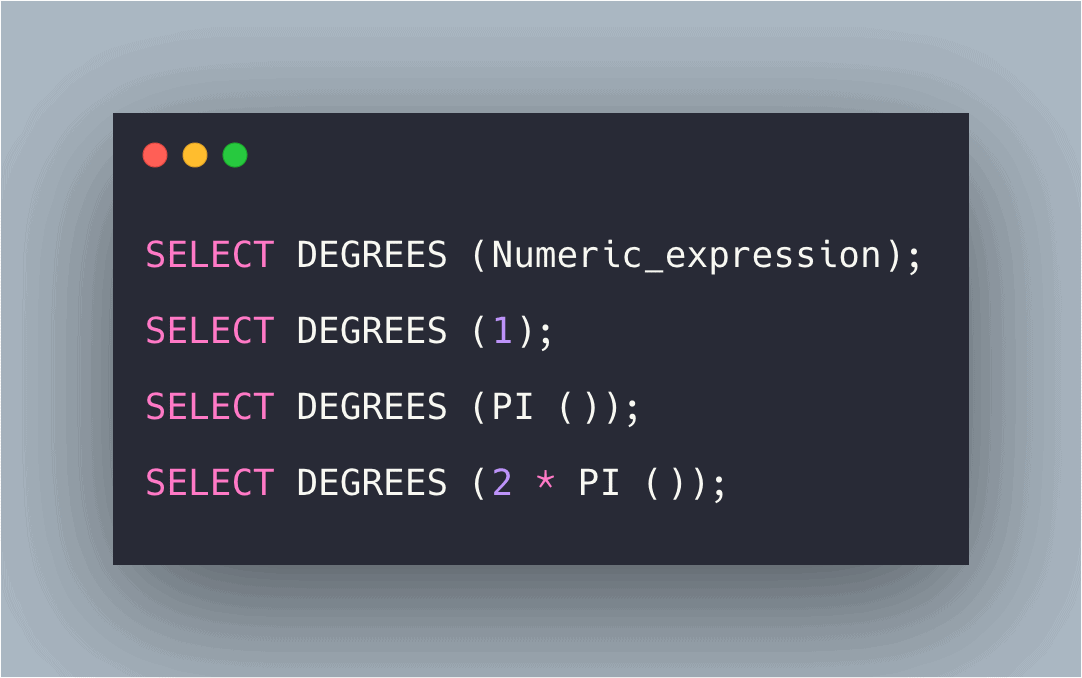# SQL DEGREES() Function: Complete Guide

0
7The SQL DEGREES() function converts a value in radians to degrees.

## SQL DEGREES()

SQL DEGREES() is a built-in function that is used to convert a value from radians to degrees. The DEGREES() function requires the number as a parameter and returns the radian values.

Here, the angle (in radians) is provided as an input to the function and the function will return the value in degrees.

### Syntax

`SELECT DEGREES (Numeric_expression);`

### Parameters

Numeric_expression: The expression whose value in degrees is to be returned.

### Example 1

```SELECT DEGREES (1);
```

`57`

### Explanation

Here, the degrees the Numeric_expression is returned.

### Example 2

```SELECT DEGREES (2.3);
```

#### Output

```131.780292880089319851
```

### Explanation

Here, the degrees of the fractional Numeric_expression is returned.

### Example 3

```SELECT DEGREES (PI ());
```

`180`

### Explanation

Here, the degree of the PI function is returned which is passed as an argument to the function.

### Example 4

`SELECT DEGREES (ASIN (0.5));`

#### Output

```30.000000000000004
```

### Explanation

Here, the degree of the ASIN function is returned whose value is passed as an argument to the function.

It works in SQL Server (starting with 2008), Azure SQL Database, Azure SQL Data Warehouse, Parallel Data Warehouse

## MySQL DEGREES() Function

MySQL DEGREES() function converts the radian value into degrees.

```SELECT DEGREES(2);
```

#### Output

`114.59155902616465`

### Example 2

```SELECT DEGREES(PI()*2);
```

`360.0`

## Conclusion

SQL DEGREES() function converts a number in radians to degrees.